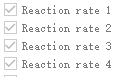# How to find the corresponding alias of reaction rate?

HI

I have two gas reactions and 2 des reactions.

It is a bit confusing when I want to watch the reaction rates, since both “vtk output” and “monitor” use the number of reaction rate.(like the above picture, I can only see reaction 1 or reaction 2, rather than the alias.)

Is it the number of reaction rates follow the order of reaction in the “.mfix” file? If there are des reactions, does it number from gas reaction and then des reaction? If the reactions are disabled, whether the numbering order will change or not?

Best Regards

1 Like

The `ReactionRate` array in MFIX is poorly named, and should be thought more generally a user output variable. MFIX does not store data in the `ReactionRate` array by default. This means that you must put data in it via a user function. Therefore, the data in `Reaction rate 1` will be whatever you stored in that array location.

so, the usr_rates subroutine and des_usr_rates subroutine, which one lies first?
Or is there any other method to record the reaction rate, and shows the des reactions and gas reactions clearly?

The array ReactionRate stores cell data so it is suitable for the usr_rates.f. See example in the TFM silane pyrolysis tutorial:

``````! Save reaction rates for visualization
IF(nrr>=RX1F) ReactionRates(IJK,RX1F) = RATES(RX1F)
IF(nrr>=RX1R) ReactionRates(IJK,RX1R) = RATES(RX1R)
IF(nrr>=RX2F) ReactionRates(IJK,RX2F) = RATES(RX2F)
IF(nrr>=RX2R) ReactionRates(IJK,RX2R) = RATES(RX2R)
IF(nrr>=RX3)  ReactionRates(IJK,RX3)  = RATES(RX3)
IF(nrr>=RX4)  ReactionRates(IJK,RX4)  = RATES(RX4)
``````

IJK is the cell index. I recommend you add a descriptive label in the GUI (Output>VTK>Reaction pane) for each reaction rate you store.

Thank you
But my question is if I have both solid phase reaction, which is defined in ”usr_des_rates.f”, and gas reaction, which is defined in ”usr_rates.f”, what is the order of numbering?

Reactions rates for the gas phase (usr_rates.f) are locally stored in RATES(:), while DES reactions rates are locally stored (for each particle, in usr_rate_des.f) in DES_RATES(:). To visualize them, you need to save them into an IJK (i.e. for each fluid cell) array ReactioRates(IJK,:). It is up to you to decide which rates to save in Reactionrates and in what order. You don’t even have to save all rates if you don’t want to. If you find this confusing, just store all gas reactions first and then store all des reactions next.

The variable `NO_OF_RXNS` tells you how many gas reactions you have, and `NO_OF_DES_RXNS` is the number of DES rates. The array ReactionRates must be allocated so it can hold the data. If you want to store `NO_OF_RXNS=5` and `NO_OF_DES_RXNS=3`, then set `ReactionRates array size` to 8 in the GUI, at the bottom on the `Model setup` pane, i.e. set nrr=8 in the .mfx file. I have seen people do the following:

bottom of user_rates.f:

``````       ReactionRates(IJK,1:NO_OF_RXNS) = RATES
``````

bottom of user_rates_des.f:

``````   lb = NO_OF_RXNS +1
ub = NO_OF_RXNS + NO_OF_DES_RXNS

ReactionRates(IJK,lb:ub) = ReactionRates(IJK,lB:uB) + DES_RATES
``````

Then at every IJK cell, you will have 8 rates: the first five are the gas phase reactions, the next 3 are the sum of the reactions for all particles in cell IJK. Again, I recommend you add a descriptive label in the GUI (Output>VTK>Reaction pane) for each reaction rate you store.

4 Likes